## Easiest Way to Understand VLOOKUP in Excel

What is VLOOKUP in Excel? How do you use it? And when should you use it? In this article, we will go through all that. And in the end we will also go through common errors you could get with the VLOOKUP function.

## What is VLOOKUP in Excel? (And its limitation)

VLOOKUP is a built-in function in Excel. This function looks for a value of your choice in a column from top to bottom and once that value is found, it returns any value to the right in that row. In other words, Excel starts in the leftmost column, top to bottom Excel looks for the lookup value and then once the value is found, it moves to the right: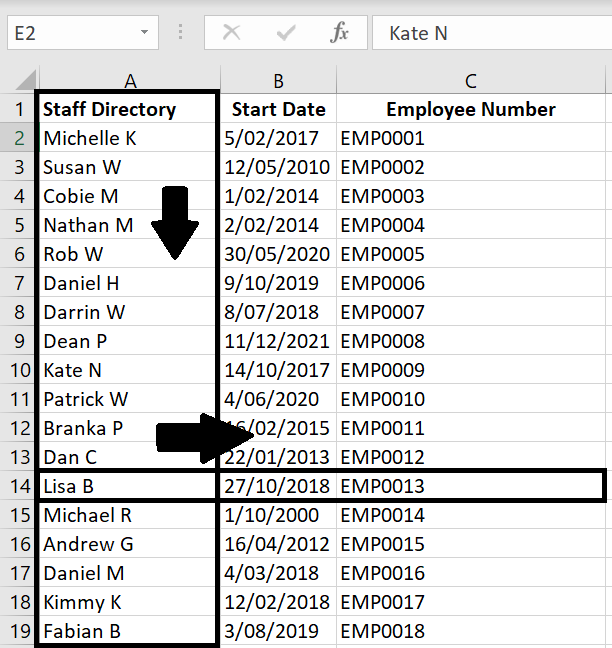The limitation with VLOOKUP is that once the value of your choice is found in a column, it can only return a value on the right-hand side of that cell. Using the example above, given the name of a staff, we can find the staff’s Start Date and Employee Number. But we will not be able to use the Employee Number or Start Date to find the staff’s name.

## How Does VLOOKUP Work?

Let’s jump straight into it. The VLOOKUP function is:

• VLOOKUP = (lookup_value, table_array, col_index_num, [range_lookup])

The easiest way to understand this is:

• Excel will look through the first column in our table of data (table_array) to find a value of our choice (lookup_value). Once found, it will move to the right to return the value in x column (col_index_num). [range_lookup] is an option we have whether we want Excel to find an exact match (FALSE) or an approximate match (TRUE) with our lookup_value. If left blank, by default it will be true.

Let’s put this in practice: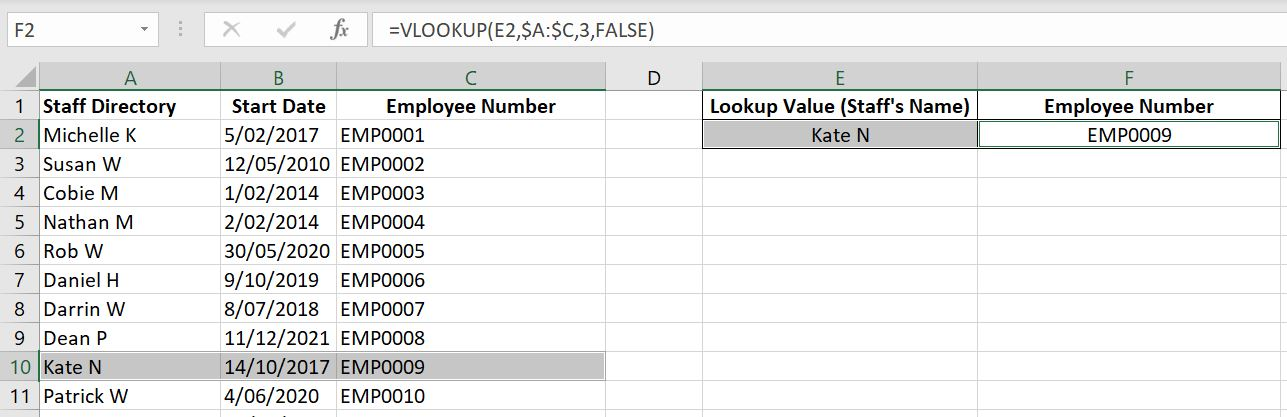With the example above: VLOOKUP(E2, \$A2:\$C, 3, FALSE):

• Excel is looking for value in E2 (“Kate N”) in the Column A (first column in table A:C). Once it finds “Kate N” which in this case is in row 10, it returns the value in the 3rd column (as we have specified). Column A is 1st column, B is 2nd column and C is 3rd column.

Note: hypothetically if the table_array is F1:L100, F would become 1st column, G becomes 2nd column, H becomes 3rd column, I becomes 4th…and so on.

If we have a range of lookup values listed down as a column or across as a row, we could enter that range as the lookup_value. In the example below, we’ve selected E2:E5 as the lookup_value and Excel automatically each lookup_value for us one by one: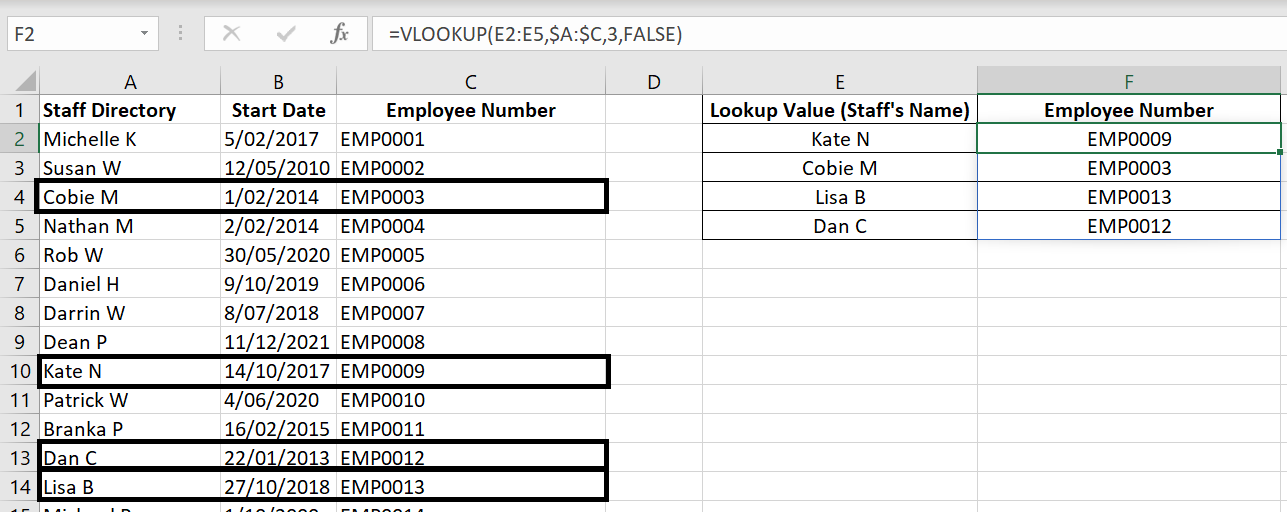But alternatively we could of course always put E2 as the lookup_value and drag the formula down. In that case, just remember to lock in the table_array with absolute referencing. Otherwise the table_array would shift: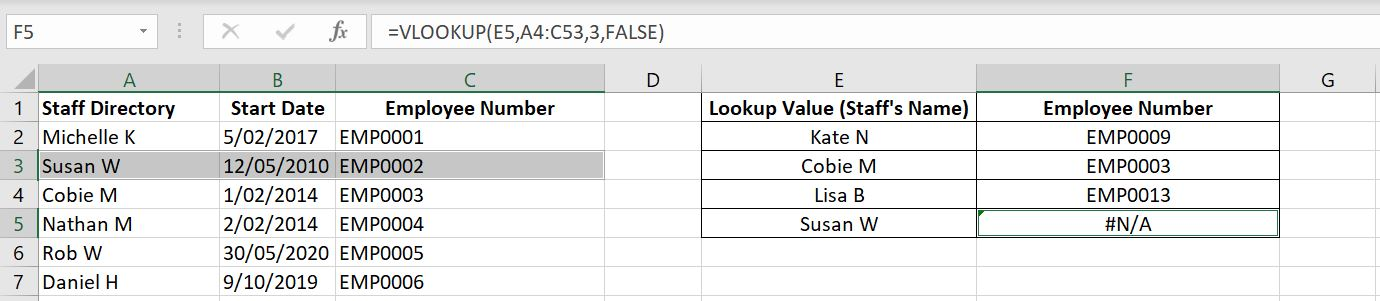Because we entered A1:C50 as the table_array in the first VLOOKUP function in F2, as I dragged the formula down to F5, the table has then shifted down one row by one row to eventually become A4:C53. But because “Susan W” is in row 3, it is no longer part of that table_array. To fix that, simply add \$ signs to the table_array so it’s fixed: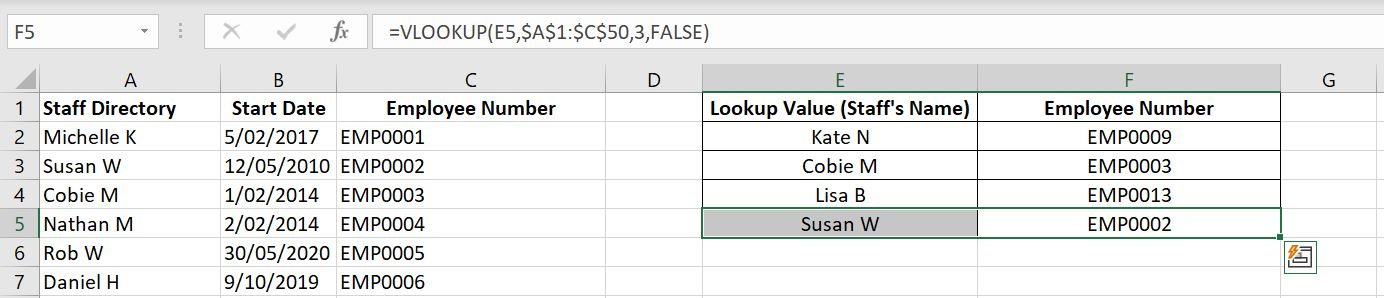As you can see, the VLOOKUP function is not too hard to use. Now let’s have a look at the common errors you could get with VLOOKUP

## Common Errors with VLOOKUP

### #NA – Cannot Find The Value

#NA is probably the most common error you would find with VLOOKUP. It simply means the lookup_value cannot be found. Here’s a few reasons why:

The lookup_value cannot be found. With the example below, there’s no “Kate” in Column A: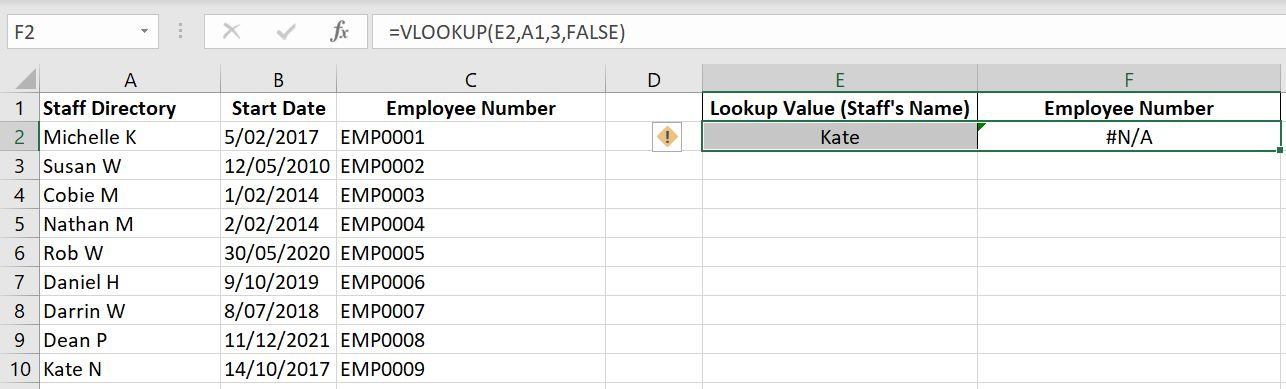The example below is not as obvious and is probably one that confuses most people because “Kate N” is clearly in Column A (row 10). The issue is that with exact match, Excel really needs the lookup_value to match the value in column A exactly. In E2, the lookup_value is actually “Kate N ” and the value in column A is “Kate N”. The space at the end, “Kate N ” means it is not the same as “Kate N”.

Note: Excel will also return #NA if we have two spaces in between “Kate” and “N” such as “Kate N”.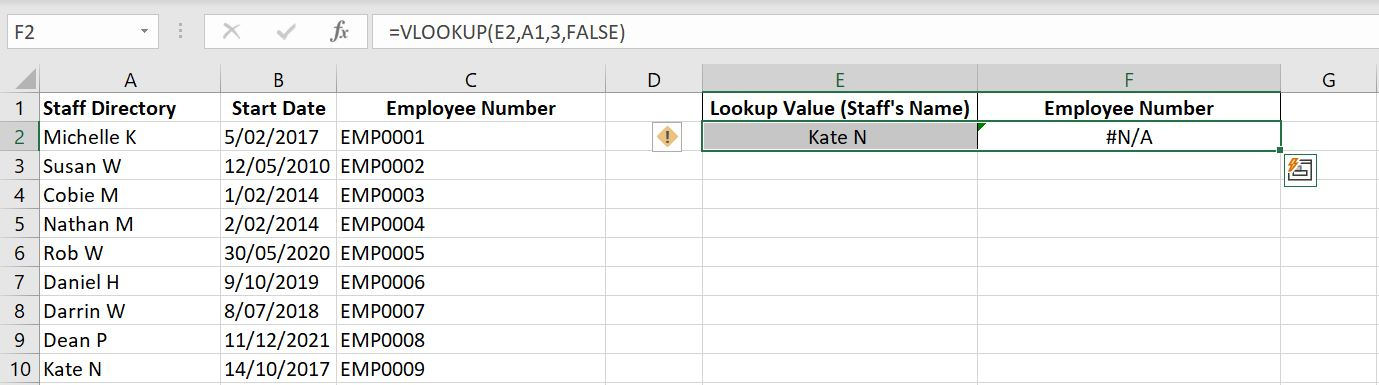In these cases, check your lookup_value to make sure it matches exactly with what is in Column A. Useful tip: you could add a dropdown list of column A in the lookup_value cell. This way you are guaranteed that the value will match. If you want to know how to add a dropdown list, check out Dropdown List in Excel.

Besides from the lookup_value, also make sure your table_array is correct. Remember Excel will only search for the lookup_value in the leftmost column in the table_array. In the example below, the table_array is A1:D100 and column A does not have “Kate N”.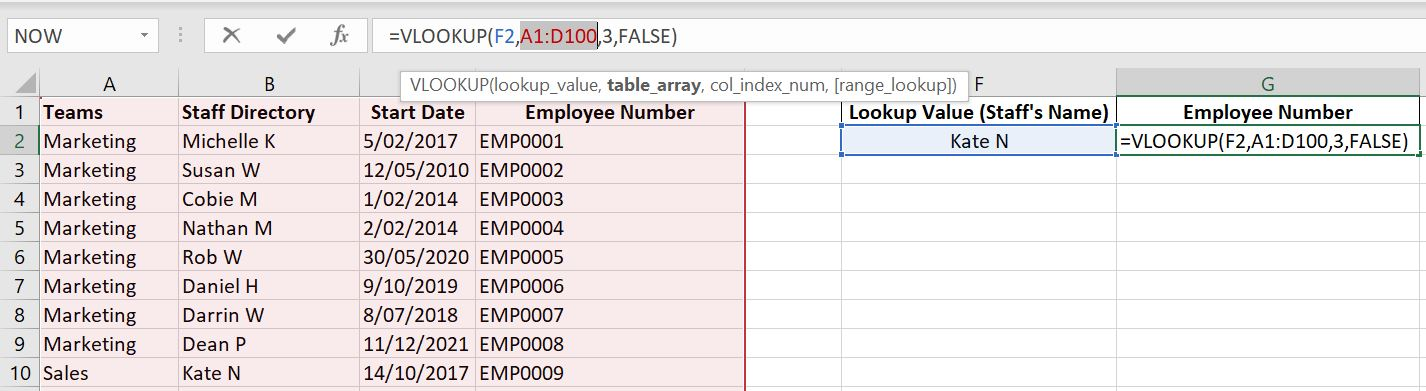In the example below as well, column B also does not have “Kate N”: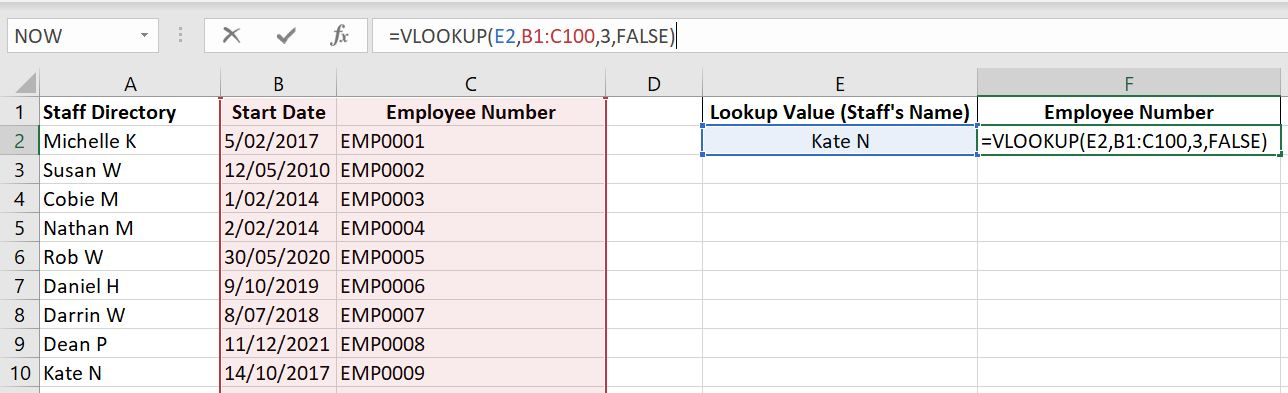### #REF – Referenced Cell is not Valid

It’s not as often that you get a #REF with VLOOKUP. With all #REF, it is because an invalid cell is referenced. This happens when col_index_num is outside the table_array.

As an example below, the table_array is A1:C100 which means Column A is col_index_num 1, Column B is col_index_num 2 and Column C is col_index_num 3. Because we only have columns A to C, when we enter 4 (or beyond) for col_index_num, a #REF is returned.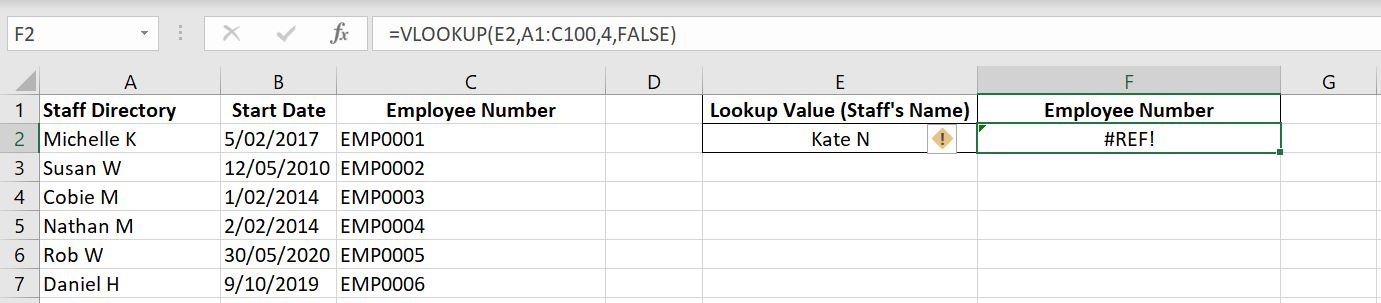### #VALUE – Unexpected Input in the VLOOKUP Function

Hopefully you will never see this. Unlike with #REF above where we put down a col_index_num beyond the table_array, this time we have gone the other way around. The first column in the table_array is col_index_num 1, the second column is col_index_num 2, third is col_index_num 3…and so on. The idea is it starts with 1 and can go to however big your table_array is. But it can never be less than 1. If you enter 0 or a negative number for col_index_num, you will get a #VALUE.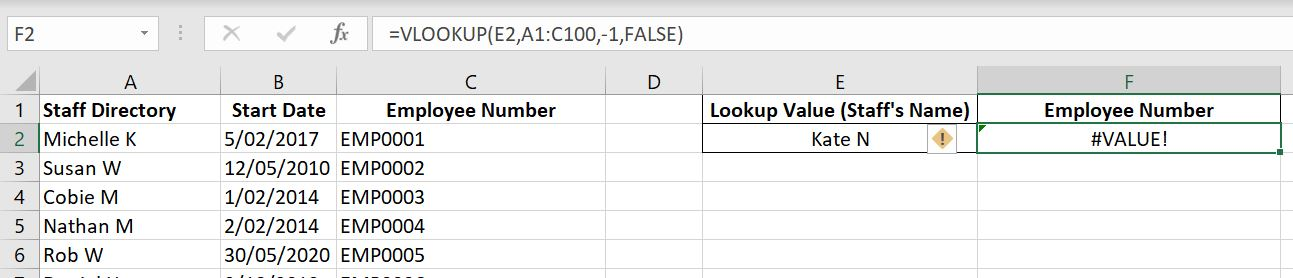We hope you now understand how the VLOOKUP function works and more importantly know when to use it and how to avoid getting an error. For any feedback on this article, please leave a comment below!